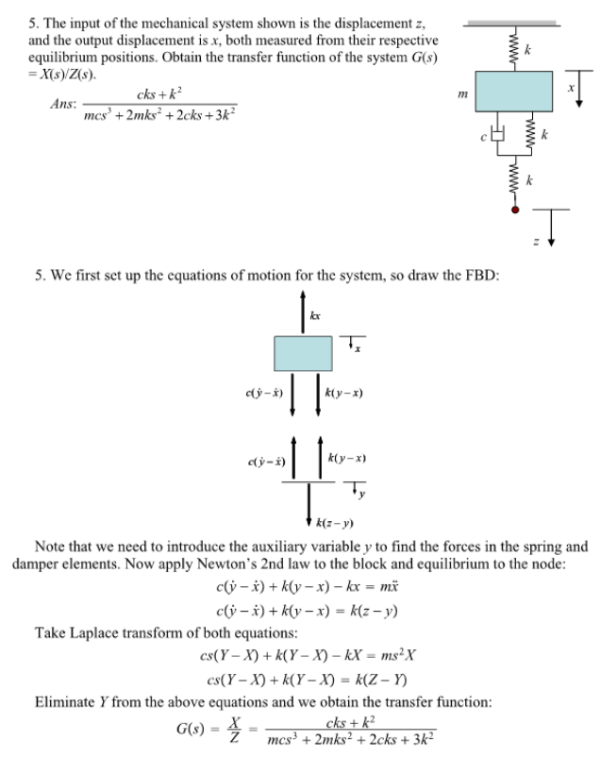The input of the mechanical system shown is the displacement z, and the output displacement is x, both measured from their respective equilibrium positions. Obtain the transfer function of the system G(s)=X(s)/Z(s).The input of the mechanical system shown is the displacement z, and the output displacement is x, both measured from their respective equilibrium positions. Obtain the transfer function of the system G(s)=X(s)/Z(s).

System Dynamics Page 2 dynamics dynamics dynamics dynamics dynamics dynamics dynamics System dynamics Page 3